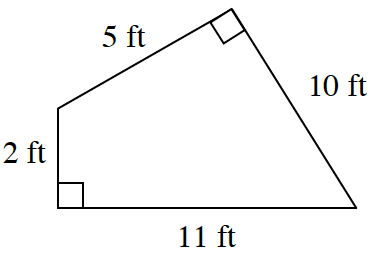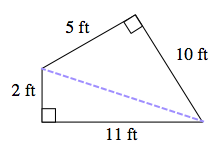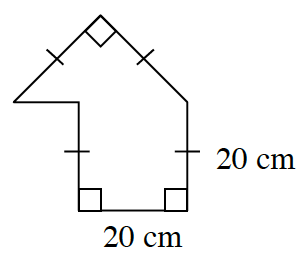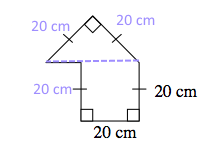### Home > CCG > Chapter 8 > Lesson 8.1.3 > Problem8-29

8-29.

Find the area and perimeter of each shape below. Show all work.

1.How can you divide this into easier shapes to find the area?area = $36 \text{ ft}^2$
Remember to calculate the perimeter as well.

1.area = $600 \text{ cm}^2$
Remember to calculate the perimeter as well.